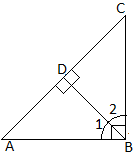# In ΔABC, if ∠B = 90°, BD ⊥ AC, then  (A) ΔADB ~ ΔBDC  (B) ΔADB ~ ΔABC  (C) ΔBDC ~ ΔABC

25 views

closed

In ΔABC, if ∠B = 90°, BD ⊥ AC, then

(C) ΔBDC ~ ΔABC

(D) All of these

+1 vote
by (56.9k points)
selected by

Correct option is (D) All of theseGiven that $\angle B=90^\circ$ & $BD\bot AC$

$\therefore$ $\angle A+\angle B+\angle C=180^\circ$   (In $\triangle ABC)$

$\Rightarrow$ $\angle A+\angle C=90^\circ$  _______________(1)   $(\because\angle B=90^\circ)$

Let $\angle ABD=\angle 1\;\&\;\angle CBD=\angle 2$

In $\triangle ADB,$

$\angle A+\angle ADB+\angle 1=180^\circ$

$\Rightarrow$ $\angle A+\angle 1=90^\circ$ _______________(2)   $(\because\angle ADB=90^\circ\,as\,BD\bot AC)$

From (1) & (2), we obtain

$\angle C=\angle1$   _______________(3)

(A) In right $\triangle ADB\;\&\;\triangle BDC,$

$\angle C=\angle1$                                      (From (3))

$\angle ADB=\angle BDC=90^\circ$               $(BD\bot AC)$

$\therefore$ $\triangle ADB\sim\triangle BDC$    (By AA similarity rule)

(B) In right $\triangle ADB\;\&\;\triangle ABC,$

$\angle DAB=\angle BAC$               (Common angle)

$\angle ADB=\angle ABC=90^\circ$                    (Given)

$\therefore$ $\triangle ADB\sim\triangle ABC$    (By AA similarity rule)

(C) $\because$ $\triangle ADB\sim\triangle BDC$ & $\triangle ADB\sim\triangle ABC$

$\therefore$ $\triangle BDC\sim\triangle ABC$

+1 vote
by (35.1k points)

Correct option is: (D) All of these